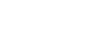Graduate Program in Electrical Engineering (PPGEE) Escola Politécnica da Universidade de São Paulo

## Fellowship Exam Program (only for Master’s and Direct Entry Doctorate Students)1 – Number Sets

1.1 – Natural numbers, integer numbers: divisibility, lowest common multiples, largest common denominators, prime factorization.

1.2 – Rational numbers and elementary notions of real numbers: operations and properties, order relations, absolute value, inequalities, percentages.

1.3 – Complex numbers: representation and operations with complex numbers in algebraic and trigonometric form, modulus of complex numbers, roots of complex numbers.

1.4 – Numerical sequences. Arithmetic and geometric progressions. Sum of an infinite number in terms of an arithmetic progression and a geometric progression. Notion of the limits of a sequence, sum of the infinite terms of a geometric progression with a common ratio modulus of less than 1. Decimal representation of a real number.2 – Planar Geometry

2.1 – Geometric planar figures: lines, rays, line segments, angles, polygons, circumferences, circles.

2.2 – Parallel and perpendicular lines in a plane. Parallel lines cut by transversals; Thales’ Theorem.

2.3 – Triangles: sum of the internal and external angles of a triangle, triangle area, triangle congruence, triangle similarity, triangle relationships, specific properties of right triangles, trigonometry of right triangles.

2.4 – Convex polygons: sum of the internal and external angles, congruence and similarity of polygons, regular polygons, the area and specific properties of trapezoids, parallelograms, rhombuses, rectangles and squares.

2.5 – Circumference and the Circle: circumference relationships, length of a circumference, area of a circle and circle sectors.

2.6 – Geometric constructions using a ruler and a compass.3 – Spatial Geometry

3.1 – Geometric spatial figures: lines and planes in space, dihedral and polyhedral angles, convex polyhedrals, regular polyhedrals.

3.2 – Relative positions of lines and planes: parallelism and perpendicularity in space, skew lines.

3.3 – Prisms, pyramids, cylinders, cones and their respective frustums: calculating their areas and volumes.

3.4 – Spheres and spherical surfaces: calculating surface areas and volumes.

3.5 – Similarity between planar and spatial figures: ratio between lengths, areas and volumes.4 – Functions

4.1 – Notions of functions. Graphs. Even and odd functions. Increasing and decreasing functions. Maximums and minimums.

4.2 – Modular functions, linear functions, affine and quadratic functions. Equations and inequations involving these functions.

4.3 – Composition of functions and inverse functions.

4.4 – Exponential functions and logarithmic functions: fundamental properties, graphs, equations and inequations involving these functions.5 – Polynomials

5.1 – Degrees of polynomials. Addition and multiplication of polynomials. Polynomial identities.

5.2 – Factoring polynomials. Algorithm to divide polynomials. Dividing polynomials by  x – a.6 – Algebraic Equations

6.2 – Relationships between coefficients and radicals. Algebraic equations with real coefficients: rational radical expressions, rewriting radicals using complex conjugates.7 – Combinations and Probability

7.1 – Counting problems.

7.2 – Multisets, permutations and combinations.

7.3 – Newton’s binomial theorem.

7.4 – Probability: notion and distribution of probabilities, conditional probability and independent events.

7.5 – Notions of Statistics: frequency distributions (averages and medians), measurements of dispersion (variances and standard deviations).8 – Linear Systems and Matrices

8.1 – Linear systems: resolution and discussion.

8.2 – Matrices: addition and multiplication of matrices and matrix inverses. Matrices associated with linear systems.

8.3 – Determinants: properties and applications for linear systems. Cramer’s rule.9 – Analytic Geometry

9.1 – Cartesian coordinates: locating points on a line and a plane using Cartesian coordinates, the distance between two points, the use of Cartesian coordinates for solving simple line and plane geometric problems.

9.2 – Study of lines in planar analytic geometry: normal form of the equation of a line, angular coefficient, parallel and perpendicular lines, two variable first degree equations and inequations, distance between a point and a line.

9.3 – Study of circumference in analytic geometry: equation, intersection of circumferences and lines, tangential lines and circumferences, intersections and tangents of circumferences.

9.4 – Analytic representation of geometric locations, definition and representation of conic sections, reduced equation of a conic section, intersection of lines and conic sections.10 – Trigonometry

10.1 – Arcs and angles: arc measurement (radians), relationship between arcs and angles.

10.2 – Trigonometric functions: definition, periodicity, parity, calculation of notable angles, graphics.

10.3 – Formulas for addition, subtraction, duplication and bisection of arcs. Transforming the sum of trigonometric functions into products.

10.4 – Basic trigonometric identities. Equations and inequations involving trigonometric functions.

10.5 – Sines and Cosines. Solving triangles.11 – Bibliography:

IEZZI, Gelson. (2004). Fundamentos de Matemática Elementar. São Paulo: Editora Atual. Volumes 1 a 11.

﻿